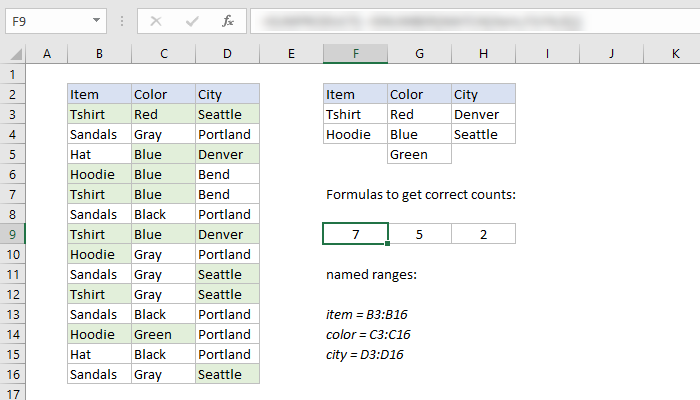## Excel

### Date Format Conversion

This Excel formula converts bogus date formats such as 19/08/2015 11:39:00 AM and 4/12/2013 10:37:58 PM into a proper Excel date. Note, that the second bogus date format was meant to be Dec 4 and not Apr 12. This formula takes care of this problem as well. Enjoy.

```=DATE(RIGHT(IF(LEN(TEXT(A2,"m/d/yyyy"))>10,LEFT(TEXT(A2,"m/d/yyyy"),FIND(" ",TEXT(A2,"m/d/yyyy"))-1),TEXT(A2,"m/d/yyyy")),4),IF(LEFT(MID(LEFT(IF(LEN(TEXT(A2,"m/d/yyyy"))>10,LEFT(TEXT(A2,"m/d/yyyy"),FIND(" ",TEXT(A2,"m/d/yyyy"))-1),TEXT(A2,"m/d/yyyy")),LEN(IF(LEN(TEXT(A2,"m/d/yyyy"))>10,LEFT(TEXT(A2,"m/d/yyyy"),FIND(" ",TEXT(A2,"m/d/yyyy"))-1),TEXT(A2,"m/d/yyyy")))-4-1),FIND("/",LEFT(IF(LEN(TEXT(A2,"m/d/yyyy"))>10,LEFT(TEXT(A2,"m/d/yyyy"),FIND(" ",TEXT(A2,"m/d/yyyy"))-1),TEXT(A2,"m/d/yyyy")),LEN(IF(LEN(TEXT(A2,"m/d/yyyy"))>10,LEFT(TEXT(A2,"m/d/yyyy"),FIND(" ",TEXT(A2,"m/d/yyyy"))-1),TEXT(A2,"m/d/yyyy")))-4-1))+1,2))="0",MID(MID(LEFT(IF(LEN(TEXT(A2,"m/d/yyyy"))>10,LEFT(TEXT(A2,"m/d/yyyy"),FIND(" ",TEXT(A2,"m/d/yyyy"))-1),TEXT(A2,"m/d/yyyy")),LEN(IF(LEN(TEXT(A2,"m/d/yyyy"))>10,LEFT(TEXT(A2,"m/d/yyyy"),FIND(" ",TEXT(A2,"m/d/yyyy"))-1),TEXT(A2,"m/d/yyyy")))-4-1),FIND("/",LEFT(IF(LEN(TEXT(A2,"m/d/yyyy"))>10,LEFT(TEXT(A2,"m/d/yyyy"),FIND(" ",TEXT(A2,"m/d/yyyy"))-1),TEXT(A2,"m/d/yyyy")),LEN(IF(LEN(TEXT(A2,"m/d/yyyy"))>10,LEFT(TEXT(A2,"m/d/yyyy"),FIND(" ",TEXT(A2,"m/d/yyyy"))-1),TEXT(A2,"m/d/yyyy")))-4-1))+1,2),2,1),MID(LEFT(IF(LEN(TEXT(A2,"m/d/yyyy"))>10,LEFT(TEXT(A2,"m/d/yyyy"),FIND(" ",TEXT(A2,"m/d/yyyy"))-1),TEXT(A2,"m/d/yyyy")),LEN(IF(LEN(TEXT(A2,"m/d/yyyy"))>10,LEFT(TEXT(A2,"m/d/yyyy"),FIND(" ",TEXT(A2,"m/d/yyyy"))-1),TEXT(A2,"m/d/yyyy")))-4-1),FIND("/",LEFT(IF(LEN(TEXT(A2,"m/d/yyyy"))>10,LEFT(TEXT(A2,"m/d/yyyy"),FIND(" ",TEXT(A2,"m/d/yyyy"))-1),TEXT(A2,"m/d/yyyy")),LEN(IF(LEN(TEXT(A2,"m/d/yyyy"))>10,LEFT(TEXT(A2,"m/d/yyyy"),FIND(" ",TEXT(A2,"m/d/yyyy"))-1),TEXT(A2,"m/d/yyyy")))-4-1))+1,2)),LEFT(LEFT(IF(LEN(TEXT(A2,"m/d/yyyy"))>10,LEFT(TEXT(A2,"m/d/yyyy"),FIND(" ",TEXT(A2,"m/d/yyyy"))-1),TEXT(A2,"m/d/yyyy")),LEN(IF(LEN(TEXT(A2,"m/d/yyyy"))>10,LEFT(TEXT(A2,"m/d/yyyy"),FIND(" ",TEXT(A2,"m/d/yyyy"))-1),TEXT(A2,"m/d/yyyy")))-4-1),FIND("/",LEFT(IF(LEN(TEXT(A2,"m/d/yyyy"))>10,LEFT(TEXT(A2,"m/d/yyyy"),FIND(" ",TEXT(A2,"m/d/yyyy"))-1),TEXT(A2,"m/d/yyyy")),LEN(IF(LEN(TEXT(A2,"m/d/yyyy"))>10,LEFT(TEXT(A2,"m/d/yyyy"),FIND(" ",TEXT(A2,"m/d/yyyy"))-1),TEXT(A2,"m/d/yyyy")))-4-1))-1))
```

### SumProductThis Excel formula counts the number of unique items in a cell range and excludes blanks:

`=SUMPRODUCT((A2:A10<>"")/COUNTIF(A2:A10,A2:A10&""))`

It can also be used instead of complicated array formulas or pivot tables, which can be very useful for certain applications.

`=SUMPRODUCT(--(A1:A10="Luke Skywalker"),--(B1:B10="West"),D1:D10)`

### SumProduct (Used to COUNT instead of SUM)One problem that comes up a lot in Excel is counting or summing based on multiple OR conditions. For example, perhaps you need to analyze data and count orders in Seattle or Denver, for items that are Red, Blue, or Green? This can be surprisingly tricky, so naturally it makes a good challenge! The answer… SumProduct can be used to COUNT cells matching multiple criteria, like so:

```=SUMPRODUCT(
ISNUMBER(MATCH(item,{"Tshirt","Hoodie"},0))*
ISNUMBER(MATCH(color,{"Red","Blue","Green"},0))*
ISNUMBER(MATCH(city,{"Denver","Seattle"},0))
)```

I like this structure because it scales easily to handle more criteria, and also works with cell references (instead of hard-coded values).

```=SUMPRODUCT(
ISNUMBER(MATCH(item,F3:F4,0))*
ISNUMBER(MATCH(color,G3:G5,0))*
ISNUMBER(MATCH(city,H3:H4,0))
)```

### Latitude and Longitude Conversion

From degrees, minutes and seconds to decimal. The formula can be used for both Lat and Long. If you would like to convert…

From: `W 114° 5' 7''`
To: `-114.0852778`

Use the following Excel formula:

`=-(VALUE(MID(D5,3,FIND("°",D5)-3))+VALUE(MID(D5,FIND("°",D5)+2,FIND("'",D5)-FIND("°",D5)-2))/60+VALUE(MID(D5,FIND("'",D5)+2,FIND("''",D5)-FIND("'",D5)-2))/60/60)`

## CSS

Some usefull CSS. HTML Encoder used for proper display.

### Dropcap the first letter of a paragraph

```<div class="dropcap"><p>Copy goes here.</p></div>

.dropcap > p:first-of-type:first-letter {
font-family: Georgia,serif;
font-size: 75px;
line-height: 65px;
float: left;
margin-bottom: -10px;
position: relative;
margin-right: 8px;
color: #333333;
}
```